Over a million developers have joined DZone.{{announcement.body}}
{{announcement.title}}

# Bayesian Learning for Statistical Classification (Part 1)

DZone 's Guide to

# Bayesian Learning for Statistical Classification (Part 1)

### In this introduction to Bayesian learning for statistic classification, get several examples of the use of Bayes’ theorem and probability in statistic classification.

· AI Zone ·
Free Resource

Comment (2)

Save
{{ articles.views | formatCount}} Views

Probability theory is one of the most fundamental tools we have for describing the universe. It is especially relevant to statistical classification and can be used to derive a multitude of important results and to inform our understanding.

The Statsbot team asked Peter Mills to help you understand probability theory, Bayes’ theorem, and how they apply to statistical classification. Together, they will allow you to derive non-obvious results that can vastly improve and simplify your classification models.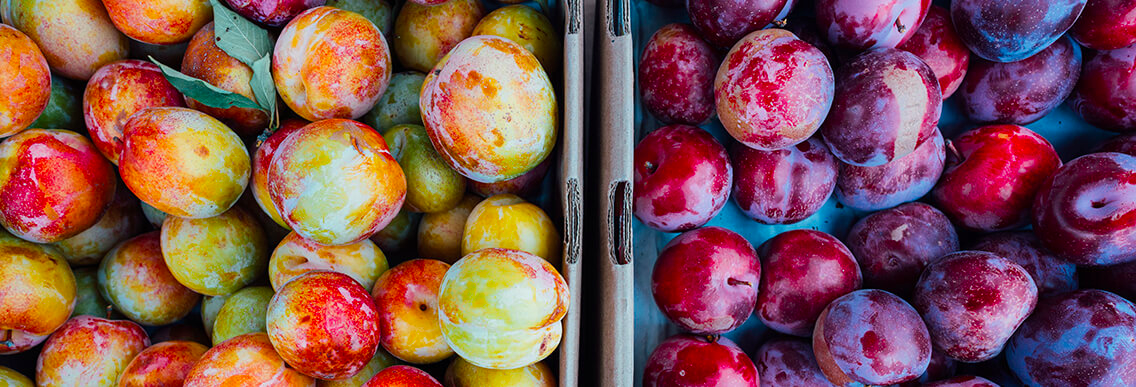This introduction to Bayesian learning for statistic classification will provide several examples of the use of Bayes’ theorem and probability in statistic classification. It will also go beyond basic probability to cover other important areas of the field, including calibration and validation.

Note that this article, while intended for beginners, nonetheless assumes knowledge of first- and some second-year university-level mathematics, especially linear algebra but also some single and multivariate calculus. If the equations seem confusing at times, try to focus instead on solving real problems.

You will learn a whole lot more about probability and statistical classification by working through some examples than just by reading about it or browsing equations. For this reason, we have prepared a set of problems at the end of the article.

## Review of Basic Probability

Suppose we roll a die. There will be six possibilities, each of which (in a fairly loaded die) will have a probability of 1/6. We can write this: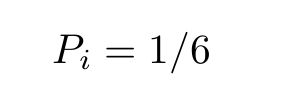...where i is the number on the top side of the die. Since at least one side will have to come up, we can also write: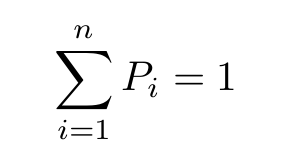Equation 1

...where n=6 is the total number of possibilities.

Now suppose we roll two dice. The joint probability of getting one of 36 pairs of numbers is given: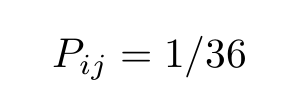...where i is the number on the first die and j that on the second.

If we ignore the number on the second die, the probability of getting a certain number (a 6, say) on the first die is given: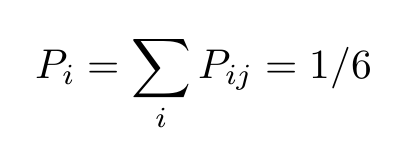Equation 2

This is known as the prior probability.

Here’s where things start getting more complicated. What is the probability of getting a certain number on one dice, given that a certain number on the other dice has come up? In this case, the two events are uncorrelated, thus the value (1/6) will always be the same. But this need not be the case.

Consider a game of Blackjack. What is the probability that the next card drawn is worth ten points (is a ten or a face card) given that the previous card was also worth ten points?

Suppose there were 7 ten-point cards out of a deck of 34 remaining before the last draw. Now the probabilities are different depending on the outcome of the previous event. If the previous card was worth ten, there is a 6/33=2/11 chance of getting a card worth ten; otherwise, the probability is 7/33.

Since the probability that the previous card was worth ten is 7/34, the joint probability, or the probability of both events occurring, is: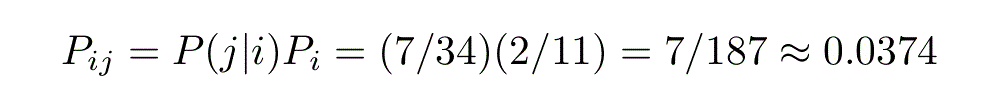...where Pi is the probability that the previous card was worth ten and P(j | i) is the conditional probability that the next card will be worth ten, given that the previous card was also worth ten.

With prior, joint, and conditional probabilities defined, we are set to write down Bayes’ theorem.

Note that these definitions are symmetric in i and j, thus: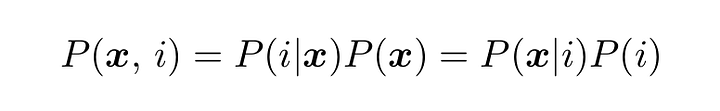Equation 3

...which is the symmetric form of Bayes’ Theorem.

## Continuous Probabilities

The extension to continuous probabilities or probability densities is straightforward. Imagine we have a continuous random variable, x, governed by a probability distribution, P(x). The probability that x takes on a value between xₒ and xₒ+dx is given: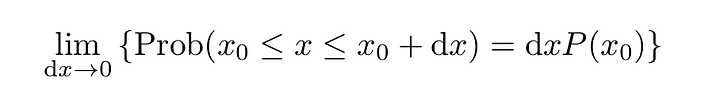Equation 4

When working with continuous random variables, summations become integrals so that Equation 2 becomes: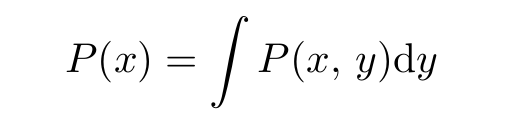Equation 5

...where P(x, y) is the joint probability of both x and y and the integral is over all of x.

In statistical classification, we are dealing with probabilities having a very specific form. One of the probabilities is scalar and discrete, while the other is vector and continuous: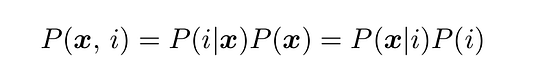Equation 6

Where i is the class or class label and x is a vector of attributes or features.

Typically, the goal of Bayesian-based statistical classification is to estimate either the joint probability, P(x, i), or the conditional probability, P(i | x). Classifications are normally done on the basis of maximum likelihood: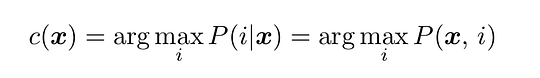Equation 7

...where c is the most likely estimate for the class; that is, the index of the largest value of the conditional probability.

Note that because P (x) is the same for a given test point, using either the joint or the conditional probability will produce the same result. The conditional probabilities of the feature space, P (x | i), are also important, as these describe the distributions of each isolated class; that is, if you remove all other class labels leaving only i, this is the distribution you are left with.

We can use the definition of probability density in Equation 4 to derive one of the oldest and most sophisticated statistical classification techniques by simply removing the limit sign. Consider picking a radius from the test point, x, then counting the number of training samples of one class or another within that distance.

The problem with this is that sometimes, the enclosed volume will contain no samples, while other times, it may contain a great many. So rather than distance, we instead fix the number of samples and implicitly choose the distance on this basis. This is what is known as a k-nearest-neighbors (KNN) classifier, where k is the number of neighboring samples used in each classification.

## Binary Classifiers

A binary classifier is special because you can, in many cases, draw a single hyperplane in the feature space that separates the two classes. A hyperplane is a subspace having dimension one less than the embedding dimension. So for a two-dimensional feature space, the boundary would be a line, while in three-dimensions, a plane.

Most binary classifiers return not an integer having only two values, but a continuous, decision function. A convenient form of the decision function would be the difference in conditional probabilities: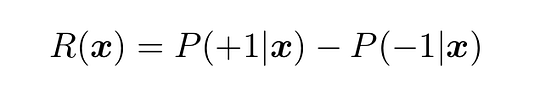Equation 8

...where, for convenience, we have chosen the class values as -1 and +1.

Unfortunately, most statistical classifiers do not return a decision function that estimates this quantity well, so a significant chunk of this article will be dedicated to describing methods of calibrating it so that it does.

Consider a pair of equally sized, one-dimensional Gaussian functions of equal width, h, and spaced an equal distance, b, from the origin. The difference in conditional probabilities is given: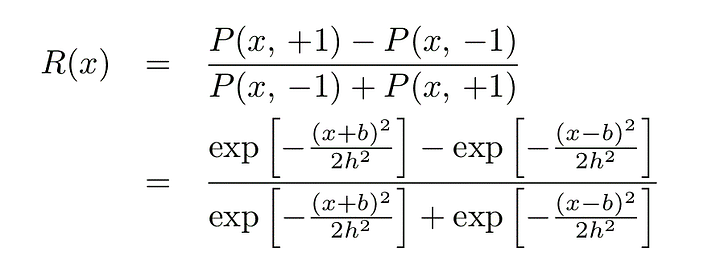...which, with some manipulation, works out to: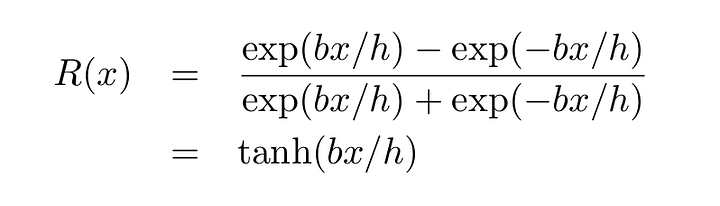Equation 9

In other words, for a pair of equal-size Gaussians, the decision function in one dimension is a hyperbolic tangent.

This may seem like a trivial example; however, the `tanh` function is found throughout the field of machine learning. In statistical classification, it is often used to correct the decision function to better estimate the conditional probabilities.

This is applied in the LIBSVM library, for instance, as well as in my own machine learning library, libAGF. The example illustrates why — the difference in conditional probabilities, R, is, more often than not, sigmoidal close to the class borders.

Consider logistic regression. In logistic regression, we use the following decision function: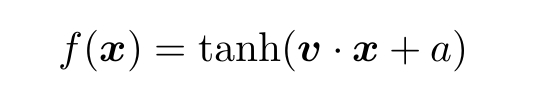Equation 10

Where v is a vector and a is a constant.

The function parameters are fitting by minimizing a cost function, for instance, a least squares: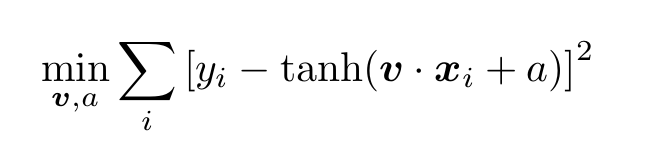Equation 11

To fit or “train” the thing, we need some training data. This comprises a set of ordered pairs of a vector in the feature space mapping onto its corresponding class value: {xᵢ : yᵢ}. Here, yᵢ takes on one of two values: -1 or +1, that is, yᵢ ∈ {-1, +1}.

The training data represents the “ground truth’’ and could be obtained in a variety of ways. Consider a land classification problem — a satellite instrument measures upwelling electromagnetic radiation in several bands and on this basis, we are interested in classifying the surface type, whether field, forest, city, or water, for instance.

The data could have been painstakingly measured by hand — an aircraft carried a terrestrial version of the instrument aloft and measured radiances, while observers in the craft noted the type of land they were flying over.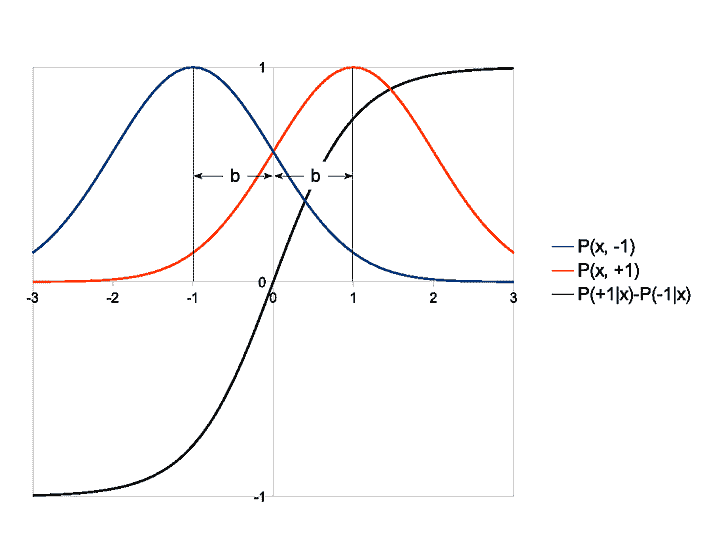It could have been modeled — perhaps we have an algorithm that we trust that returns modeled radiances depending on different parameters describing the land surface. In this case, the resulting training data is potentially infinite, although not necessarily all that accurate.

Or perhaps it was measured by the actual instrument but classified by hand. You have a simple app that brings up an image and each pixel can be classified with a mouse click on the basis of color.

Equations 10 and 11 provide a succinct illustration of the entire process of statistical classification. There is a training phase, given by 11, in which a model is derived. In this case, the model consists of a small set of function parameters which makes this an exercise in parametric statistics.

Contrast this with a non-parametric statistical model, such as KNN, which uses all of the training data for each classification. For the logistic classifier, the fitting will be nonlinear, another common technique in machine learning.

Nonlinear optimization is normally performed with an iterative, numerical algorithm, assuming the problem cannot be reduced to a closed-form, analytic solution. It is in itself a broad and diverse field, so we won’t go into the exact details. See the problem set for more info.

The model is then applied to classify a series of test points using Equation 10.

Well, that's it for Part 1! In Part 2, we'll talk more about calibration, validation, and multi-class classification.

Topics:
machine learning ,bayesian ,ai ,statistics ,neural networks ,probability ,tutorial

Comment (2)

Save
{{ articles.views | formatCount}} Views

Published at DZone with permission of

Opinions expressed by DZone contributors are their own.